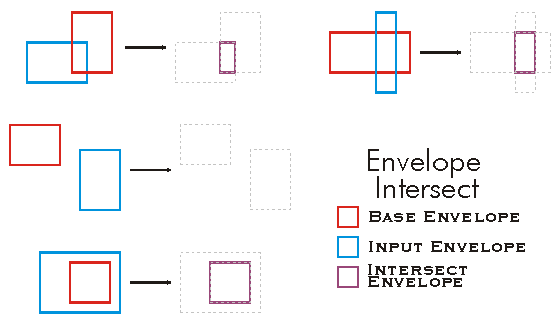ArcObjects Library Reference (Geometry)

# IEnvelope.Intersect Method

Adjusts to include only the area also included by inEnvelope.

```[Visual Basic .NET]
Public Sub Intersect ( _
ByVal inEnvelope As IEnvelope _
)```
```[C#]
public void Intersect (
IEnvelope inEnvelope
);```
```[C++]
HRESULT Intersect(
IEnvelope* inEnvelope
);```
```[C++]
Parameters
inEnvelope
inEnvelope is a parameter of type IEnvelope
```

#### Product Availability

Available with ArcGIS Engine, ArcGIS Desktop, and ArcGIS Server.

#### Description

Sets the Envelope equal to the intersection of the base Envelope and the input Envelope.  The XMin and YMin of the resulting Envelope is the maximum XMin and YMin respectively between the base and input Envelopes, and the XMax and YMax of the resulting Envelope is the minimum XMax and YMax respectively between the base and input Envelopes.  If the resulting XMin > XMax, or YMin > YMax, then the Envelope is Empty (and all XMin, XMax, YMin, and YMax are undefined.).

#### Remarks[C#]

public void Intersect()
{
IEnvelope envelope1 = new EnvelopeClass();
IEnvelope envelope2 = new EnvelopeClass();
envelope1.PutCoords(100, 100, 200, 200);
envelope2.PutCoords(150, 150, 250, 250);
envelope1.Intersect(envelope2);
IPoint lowerLeft = envelope1.LowerLeft;
IPoint lowerRight = envelope1.LowerRight;
IPoint upperLeft = envelope1.UpperLeft;
IPoint upperRight = envelope1.UpperRight;
String report = "LowerLeft  X = " + lowerLeft.X + "\n" +
"LowerLeft  Y = " + lowerLeft.Y + "\n\n" +
"LowerRight X =  " + lowerRight.X + "\n" +
"LowerRight Y =  " + lowerRight.Y + "\n\n" +
"UpperLeft  X = " + upperLeft.X + "\n" +
"UpperLeft  Y = " + upperLeft.Y + "\n\n" +
"UpperRight X =  " + upperRight.X + "\n" +
"UpperRight Y =  " + upperRight.Y;

System.Windows.Forms.MessageBox.Show(report);
}

[Visual Basic .NET]

'The example shows how to intersect 2 envelopes. The result is put in the first envelope.
Public Sub t_EnvIntersect()
Dim pEnv1 As IEnvelope
Dim pEnv2 As IEnvelope
pEnv1 = New Envelope
pEnv2 = New Envelope
pEnv1.PutCoords(100, 100, 200, 200)
pEnv2.PutCoords(150, 150, 250, 250)

pEnv1.Intersect(pEnv2)

Dim dXmin As Double, dYmin As Double, dXmax As Double, dYmax As Double
pEnv1.QueryCoords(dXmin, dYmin, dXmax, dYmax)

If pEnv1.IsEmpty Then
MsgBox("envelope is empty")
Else
MsgBox(dXmin & "," & dYmin & "," & dXmax & "," & dYmax)
End If
End Sub